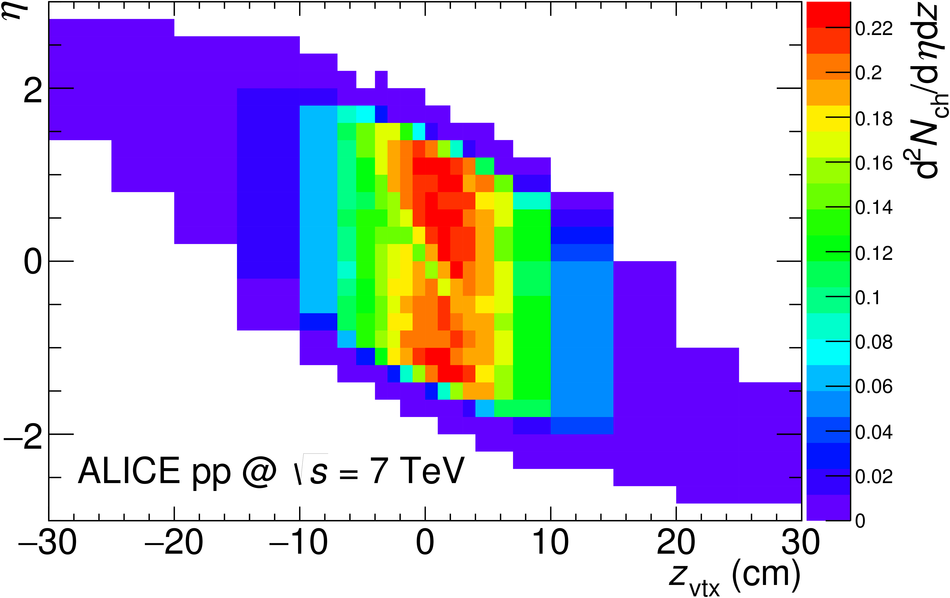# Figure 1

 For a data sample of events fulfilling the MB$_{\rm{OR}}$ trigger selection, at $\sqrt{s} = 7$ TeV, the distribution of the quantity ${\rm d}^2\nch$/${\rm d}\eta{\rm d}z$ is plotted for tracklets, in the plane pseudorapidity ($\eta$) vs. $z$ position of the SPD vertex ($z_{\rm vtx}$), showing the dependence of the $\eta$ acceptance on $z_{\rm vtx}$.